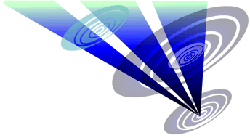Reconstruction of the 2016 Sparticles Wood formation 1.Draw a circle. Draw and extend the horizontal and vertical centerlines. 2.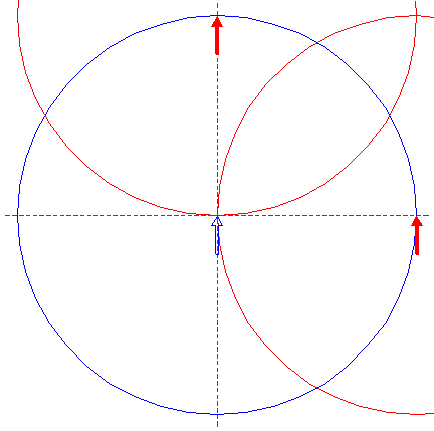Copy circle 1 two times, to its lefthand intersection with the horizontal, and to its upper intersection with the vertical centerline. 3.Draw the connecting line between the mutual intersections of circles 2. 4.Construct the inscribed square of circle 1, with one angular point on line 3. 5.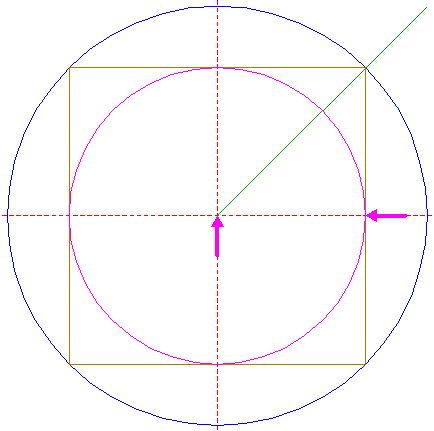Construct the inscribed circle of square 4.Steps 4 and 5 are repeated seven times, each time for the previous square and circle. 6.Construct the inscribed square of circle 5, with one angular point on line 3. 7.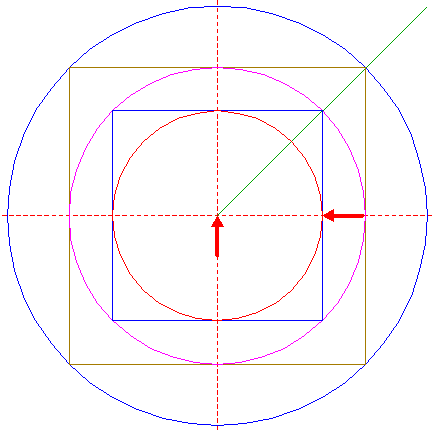Construct the inscribed circle of square 6. 8.Construct the inscribed square of circle 7, with one angular point on line 3. 9.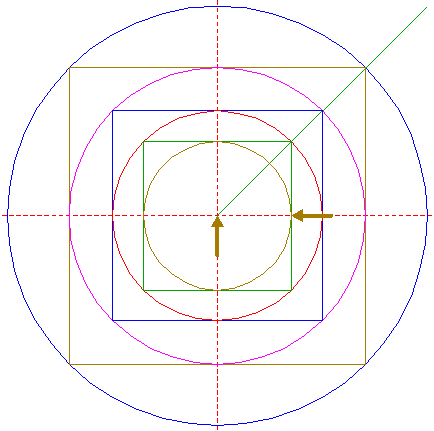Construct the inscribed circle of square 8. 10.Construct the inscribed square of circle 9, with one angular point on line 3. 11.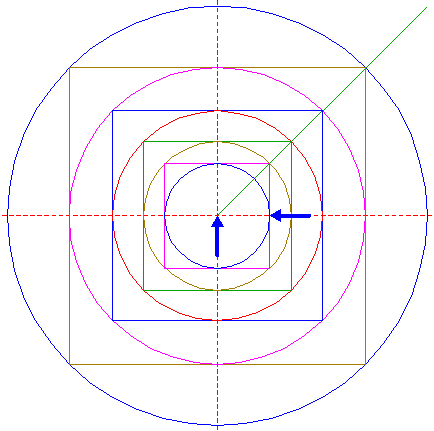Construct the inscribed circle of square 10. 12.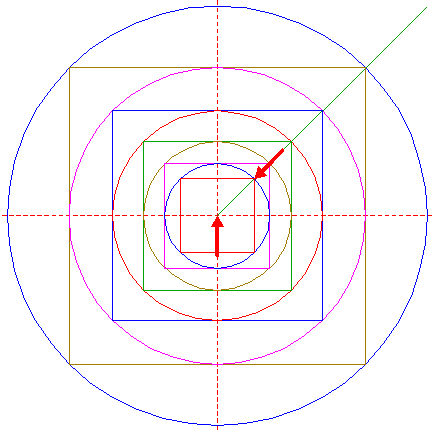Construct the inscribed square of circle 11, with one angular point on line 3. 13.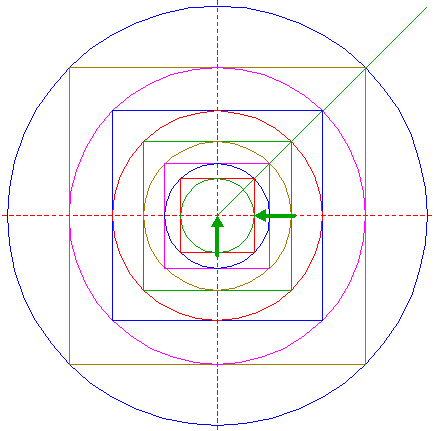Construct the inscribed circle of square 12. 14.Construct the inscribed square of circle 13, with one angular point on line 3. 15.Construct the inscribed circle of square 14. 16.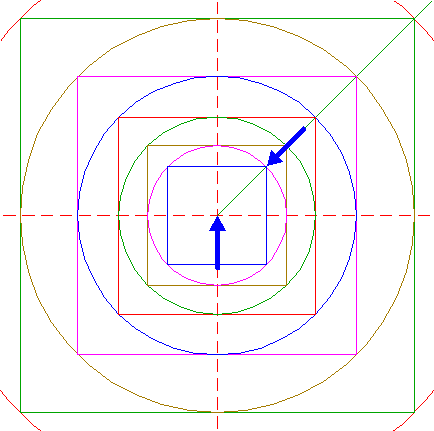Construct the inscribed square of circle 15, with one angular point on line 3. 17.Construct the inscribed circle of square 16. 18.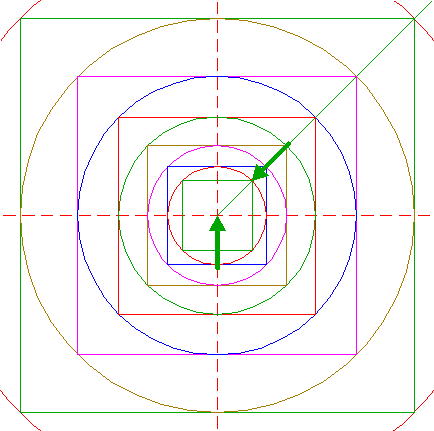Construct the inscribed square of circle 17, with one angular point on line 3. 19.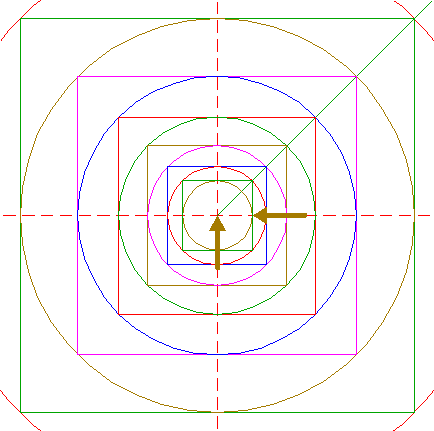Construct the inscribed circle of square 18. 20.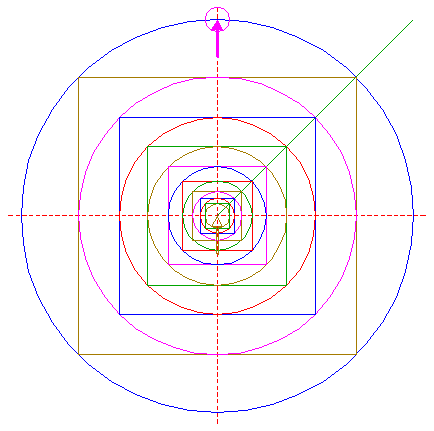Copy circle 19 to the upper intersection of circle 1 and the vertical centerline. 21.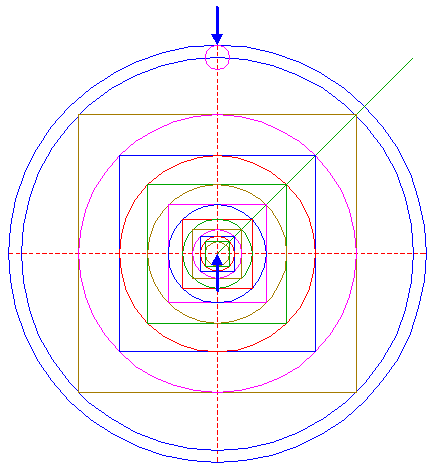Construct a circle concentric to circle 1, tangent to circle 20 at the upper side. 22.Draw four rays of circle 1, from the center to its intersections with the horizontal and vertical centerlines. 23.Circles 1 and 21, squares 4, 6, 8, 10, 12, and 14, and lines 22 are used for the final reconstruction. 24.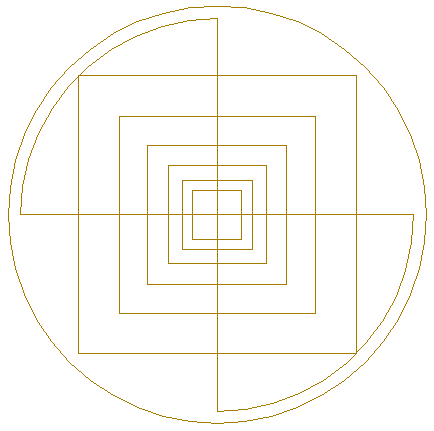Remove all parts not visible within the formation itself. 25.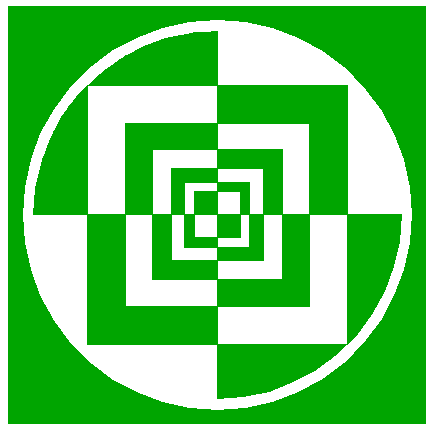Colour all areas corresponding to standing... 26....or to flattened crop, and finish the reconstruction of the 2016 Sparticles Wood formation. 27.The final result, matched with the aerial image.